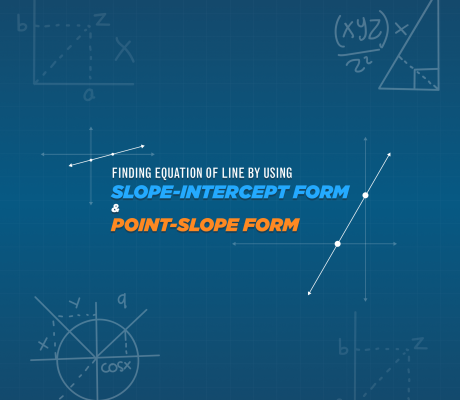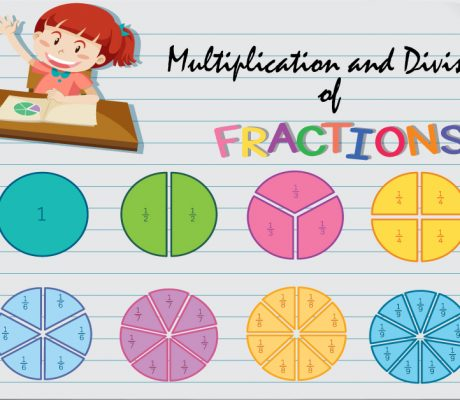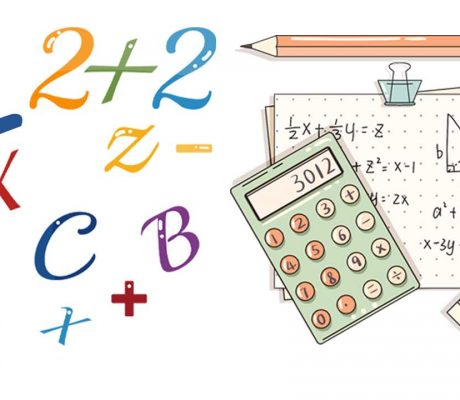### Finding The Equation Of The Line By Using The Slope-Intercept Form And Point-Slope Form

In mathematics and physics, equations of the line are used widely. Equation of the line is very essential in different branches of science and everyday problems. It is that equation in which the degree of each variable is one. This article will discuss the Slope-Intercept And Point-Slope of Linear Equations The equation of the line […]### Equivalent Fractions

Equivalent fractions are fractions that represent the same size even if the numbers look different. Let’s take a look at the pizzas below. The two fractions have different numerators and denominators, but they represent the same size of pizza slices. when simplified, is actually . It is important to remember that these two fractions still […]### Multiplying and Dividing Fractions

The truth is multiplying and dividing fractions are easier than adding and subtracting fractions. It does not require a lot of steps because it only involves multiplying and simplifying.  When multiplying fractions, you take the numerators and multiply them. You also do the same with the denominators. If needed, the final product is then simplified […]### Learn ZOE Math Practice Questions with Answers

In this blog, there are 21 math practice questions that Learn ZOE teachers prepared for you. The questions are based on different grade levels and once the answer is revealed, there will be a detailed explanation of how we got the correct answer. Feel free to answer the math practice questions first before revealing the […]